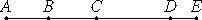# Proposition 21

If as many even numbers as we please are added together, then the sum is even.

Let as many even numbers as we please, AB, BC, CD, and DE, be added together.I say that the sum AE is even.

VII.Def.6

Since each of the numbers AB, BC, CD, and DE is even, therefore each has a half part, so that the sum AE also has a half part. But an even number is that which is divisible into two equal parts, therefore AE is even.

Therefore, if as many even numbers as we please are added together, then the sum is even.

Q.E.D.

## Guide

With this proposition, Euclid commences the study of even and odd numbers. The study continues through proposition IX.34. The statements of these propositions probably constitute the oldest part of the Elements and date back to the Pythagoreans. Indeed, their proofs depend on no other propositions (except IX.31 which discusses prime numbers and may have been inserted among these propositions because it involves odd numbers), so that the statements together with the proofs may be the oldest part of the Elements.

#### Commutativity and associativity of addition

The proof of this proposition implicitly relies on a principle that the order that numbers are summed is irrelevant. For example, when showing that the sum of the two even numbers a and b is even, first a is divided into two equal parts, a = c + c, and b is divided into two equal parts, b = d + d, therefore

a + b = (c + c) + (d + d).

But to reach the conclusion that a + b is divisible into two equal parts, we need

a + b = (c + d) + (c + d),

which adds the terms in a different order.

Of course the order that the terms are added has no effect on the sum. That is an implicit assumption made by Euclid and most everyone after him until the 19th century.

The modern way to deal with this question is to recognize two properties of addition of numbers. One of them is commutativity. Addition is commutative since for any two numbers a and b,

a + b = b + a.

Commutativity says we can two numbers in any order and get the same result.

The other property, associativity, is more subtle. When computing the sum a + b + c of three numbers, there is still a choice of which numbers to add first. You can either add a + b first to get d, then add d + c to get the sum, or you can add b + c first to get e, then add a + e to get the sum. The same sum should result. As an equation, associativity says you can move the parentheses around:

(a + b) + c = a + (b + c).

These two properties, commutativity and associativity, are enough to guarantee that when you add any number of terms together, the order that they’re added is irrelvant.

These properties should either be taken as postulates about numbers, or else proven from more basic assumptions. Besides these properties of addition, Euclid missed some other basic properties of the arithmetic operations.

#### Use of this proposition

This proposition is used in the next two and in IX.28.